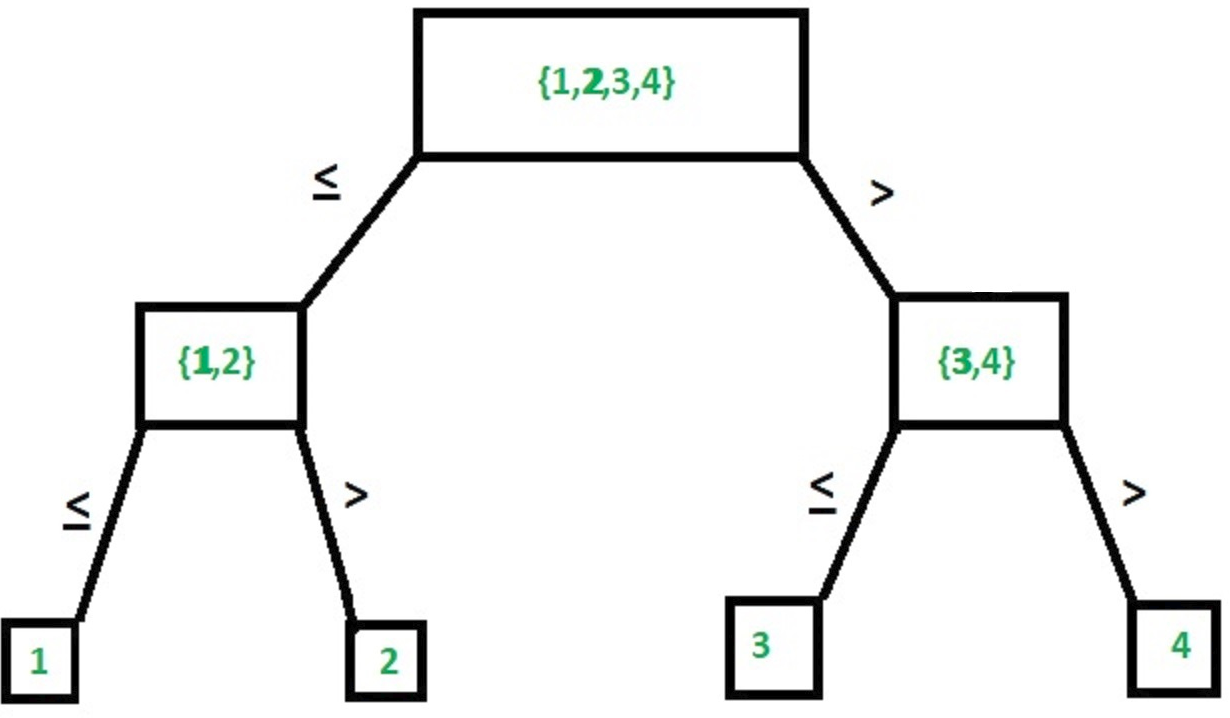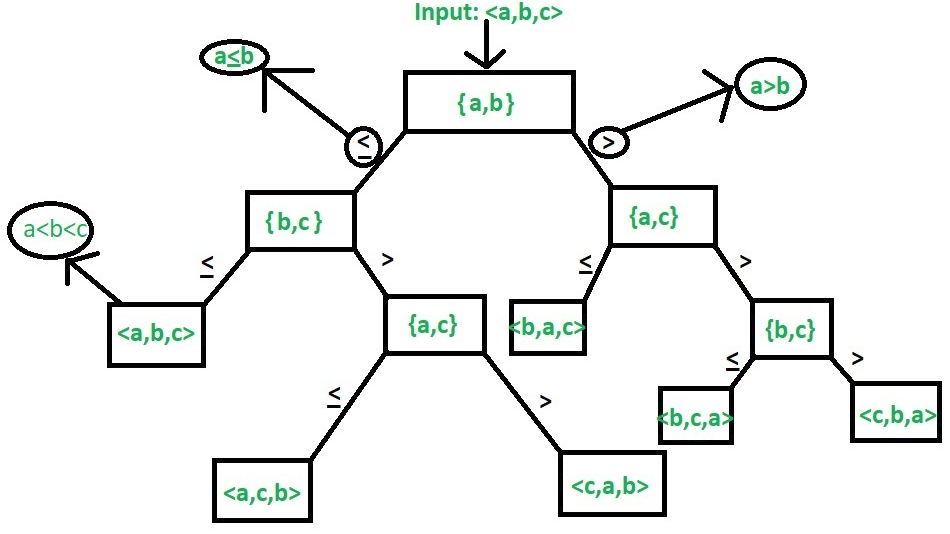GeeksforGeeks App
Open AppBrowser
Continue

Lower and upper bound theory is a mathematical concept that involves finding the smallest and largest possible values for a quantity, given certain constraints or conditions. It is often used in optimization problems, where the goal is to find the maximum or minimum value of a function subject to certain constraints.

1. In mathematical terms, the lower bound of a set of numbers is the smallest number in the set, while the upper bound is the largest number. If a set has a lower and upper bound, it is said to be bounded.
2. Lower and upper bound theory can also be used to determine the range of possible values for a variable. For example, if we know that a certain variable must be between 0 and 1, we can say that its lower bound is 0 and its upper bound is 1.
3. The concept of lower and upper bounds is closely related to the concepts of infimum and supremum. The infimum of a set is the greatest lower bound of the set, while the supremum is the least upper bound of the set.
4. Overall, lower and upper bound theory is an important tool in mathematical analysis, optimization, and decision-making, as it allows us to determine the range of possible values for a quantity and identify the optimal value within that range.

The Lower and Upper Bound Theory provides a way to find the lowest complexity algorithm to solve a problem. Before understanding the theory, first, let’s have a brief look at what Lower and Upper bounds are.

• Lower Bound –
Let L(n) be the running time of an algorithm A(say), then g(n) is the Lower Bound of A if there exist two constants C and N such that L(n) >= C*g(n) for n > N. Lower bound of an algorithm is shown by the asymptotic notation called Big Omega (or just Omega).

• Upper Bound –
Let U(n) be the running time of an algorithm A(say), then g(n) is the Upper Bound of A if there exist two constants C and N such that U(n) <= C*g(n) for n > N. Upper bound of an algorithm is shown by the asymptotic notation called Big Oh(O) (or just Oh).

1. Lower Bound Theory:
According to the lower bound theory, for a lower bound L(n) of an algorithm, it is not possible to have any other algorithm (for a common problem) whose time complexity is less than L(n) for random input. Also, every algorithm must take at least L(n) time in the worst case. Note that L(n) here is the minimum of all the possible algorithms, of maximum complexity.
The Lower Bound is very important for any algorithm. Once we calculated it, then we can compare it with the actual complexity of the algorithm and if their order is the same then we can declare our algorithm as optimal. So in this section, we will be discussing techniques for finding the lower bound of an algorithm.

Note that our main motive is to get an optimal algorithm, which is the one having its Upper Bound the Same as its Lower Bound (U(n)=L(n)). Merge Sort is a common example of an optimal algorithm.

Trivial Lower Bound –
It is the easiest method to find the lower bound. The Lower bounds which can be easily observed based on the number of input taken and the number of output produced are called Trivial Lower Bound.

Example: Multiplication of n x n matrix, where,

```Input: For 2 matrices we will have 2n2 inputs
Output: 1 matrix of order n x n, i.e.,  n2 outputs ```

In the above example, it’s easily predictable that the lower bound is O(n2).

Computational Model –
The method is for all those algorithms that are comparison-based. For example, in sorting, we have to compare the elements of the list among themselves and then sort them accordingly. Similar is the case with searching and thus we can implement the same in this case. Now we will look at some examples to understand its usage.

Ordered Searching –
It is a type of searching in which the list is already sorted.
Example-1: Linear search
Explanation –
In linear search, we compare the key with the first element if it does not match we compare it with the second element, and so on till we check against the nth element. Else we will end up with a failure.

Example-2: Binary search
Explanation –
In binary search, we check the middle element against the key, if it is greater we search the first half else we check the second half and repeat the same process.
The diagram below there is an illustration of binary search in an array consisting of 4 elementsCalculating the lower bound: The max no of comparisons is n. Let there be k levels in the tree.

1. No. of nodes will be 2k-1
2. The upper bound of no of nodes in any comparison-based search of an element in the list of size n will be n as there are a maximum of n comparisons in worst case scenario 2k-1
3. Each level will take 1 comparison thus no. of comparisons k≥|log2n|

Thus the lower bound of any comparison-based search from a list of n elements cannot be less than log(n). Therefore we can say that Binary Search is optimal as its complexity is Θ(log n).

Sorting –
The diagram below is an example of a tree formed in sorting combinations with 3 elements.Example – For n elements, finding lower bound using computation model.

Explanation –
For n elements, we have a total of n! combinations (leaf nodes). (Refer to the diagram the total combinations are 3! or 6) also, it is clear that the tree formed is a binary tree. Each level in the diagram indicates a comparison. Let there be k levels => 2k is the total number of leaf nodes in a full binary tree thus in this case we have n!≤2k.

As the k in the above example is the no of comparisons thus by computational model lower bound = k.

```Now we can say that,
n!≤2T(n)
Thus,
T(n)>|log n!|
=> n!<=nn
Thus,
log n!<=log nn
Taking ceiling function on both sides, we get
|-log nn-|>=|-log n!-|
Thus complexity becomes Θ(lognn) or Θ(nlogn) ```

Using Lower bond theory to solve the algebraic problem:

• Straight Line Program –
The type of program built without any loops or control structures is called the Straight Line Program. For example,

## C

 `//summing to nos``Sum(a, b)``{``    ``//no loops and no control structures``    ``c:= a+b;``    ``return` `c;``}`

• Algebraic Problem –
Problems related to algebra like solving equations inequalities etc. come under algebraic problems. For example, solving equation ax2+bx+c with simple programming.

## C

 `Algo_Sol(a, b, c, x)``{``    ``//1 assignment``    ``v:=a*x;` `    ``//1 assignment``    ``v:=v+b;` `    ``//1 assignment``    ``v:=v*x;` `    ``//1 assignment``    ``ans:=v+c;``    ``return` `ans;``}   `

The complexity for solving here is 4 (excluding the returning).
The above example shows us a simple way to solve an equation for a 2-degree polynomial i.e., 4 thus for nth degree polynomial we will have a complexity of O(n2).

Let us demonstrate via an algorithm.

Example: x+a0 is a polynomial of degree n.

## C

 `pow``(x, n)``  ``{``    ``p := 1;``  ` `    ``//loop from 1 to n``    ``for` `i:=1 to n``        ``p := p*x;` `    ``return` `p;``  ``}` `polynomial(A, x, n)``  ``{``     ``int` `p, v:=0;``     ``for` `i := 0 to n``  ` `         ``//loop within a loop from 0 to n``         ``v := v + A[i]*``pow``(x, i);``     ``return` `v;``  ``}`

Loop within a loop => complexity = O(n2);
Now to find an optimal algorithm we need to find the lower bound here (as per lower bound theory). As per Lower Bound Theory, The optimal algorithm to solve the above problem is the one having complexity O(n). Let’s prove this theorem using lower bounds.

Theorem: To prove that the optimal algo of solving a n degree polynomial is O(n)
Proof: The best solution for reducing the algo is to make this problem less complex by dividing the polynomial into several straight-line problems.

```=> anxn+an-1xn-1+an-2xn-2+...+a1x+a0
can be written as
((..(anx+an-1)x+..+a2)x+a1)x+a0
Now, the algorithm will be as,
v=0
v=v+an
v=v*x
v=v+an-1
v=v*x
...
v=v+a1
v=v*x
v=v+a0 ```

## C

 `polynomial(A, x, n)``     ``{``      ``int` `p, v=0;` `      ``// loop executed n times``      ``for` `i = n to 0``             ``v = (v + A[i])*x;` `         ``return` `v;``      ``}`

The complexity of this code is O(n). This way of solving such equations is called Horner’s method. Here is where lower bound theory works and gives the optimum algorithm’s complexity as O(n).
2. Upper Bound Theory:
According to the upper bound theory, for an upper bound U(n) of an algorithm, we can always solve the problem at most U(n) time. Time taken by a known algorithm to solve a problem with worse case input gives us the upper bound.

It’s difficult to provide a comprehensive list of advantages and disadvantages of lower and upper bound theory, as it depends on the specific context in which it is being used. However, here are some general advantages and disadvantages:

1. Provides a clear understanding of the range of possible values for a quantity, which can be useful in decision-making.
2. Helps to identify the optimal value within the range of possible values, which can lead to more efficient and effective solutions to problems.
3. Can be used to prove the existence of solutions to optimization problems.
4. Provides a theoretical framework for analyzing and solving a wide range of mathematical problems.